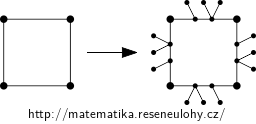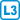## Exact number of automorphisms

For each natural number $$n$$ construct a graph $$G_n$$ that has exactly $$n$$ automorphisms, i.e. isomorphisms $$G_n\to G_n$$.

• #### Solution

$$K_1$$ has one automorphism, $$K_2$$ has two.

The cycle $$C_k$$ has $$2k$$ automorphisms $$f$$– we have $$k$$ choices for $$f(v_1)$$ and then we can assign $$f(v_2)$$ to one of the two neighbors of $$f(v_1)$$. Then the remaining vertices are uniquely determined. This construction solves the problem for all even $$n=2k$$.

We can construct graphs for odd $$n$$ using a similar principle, but we must replace the edges of $$C_n$$ with more complicated graphs so that $$f(v_2)$$ will be uniquely determined by the choice of $$f(v_1)$$. We can achieve this e.g. by replacing each edge with a path of length three, where we add 1 leaf to the first interior vertex and two leaves to the second such vertex; see the picture below.If the original cycle has vertices $$v_1,…,v_k$$ in clockwise order, then in the transformed graph every automorphism maps the vertex $$v_1$$ to some vertex of degree two. Then the image of $$v_2$$ is uniquely determined: it is the first vertex of degree two that follows $$f(v_1)$$ in a clockwise direction.

There exist other similar constructions.